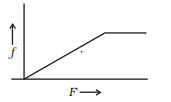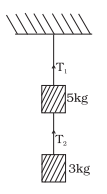# Important Questions for Laws Of Motion

In this page we have Important Questions for Laws Of Motion for Class 11 Physics . Hope you like them and do not forget to like , social share and comment at the end of the page.

Question 1
A man stands in a lift going downward with uniform velocity. He experiences a loss of weight at the start but not when lift is in uniform motion. Explain why?

The apparent weight is given by R=W-ma=mg-ma or R=m(g-a) Since lift is in acceleration in the start, a?0, so R

Question 2
Why are the wheels of vehicles are provided with mudguards?

When the wheel rotates at a high speed, the mud sticking to the wheel flies off tangentially, this is due to inertia of direction. In order that the flying mud does not spoil the clothes of passersby, the wheels are provided with mudguards.

Question 3
In a tug of war, the team that pushes harder against the ground wins. Why?

The team that pushes harder against ground gets greater reactional force and this leads them to win.

Question 4
How does friction help us in walking?

Due to friction, we are able to push the ground backward during walking. The reaction of the ground helps us to move forward.

Question 5
An astronaut accidentally gets thrown out of his small spaceship accelerating in inter-stellar space at the constant rate of 100 m/s2. What is the acceleration of the astronaut the instaant after he is outside the spaceship?

There is no external force on the astronaut when he is outside the space ship. Here we assume there is no nearby stars to exert gravitational force on him. So from second law of motion acceleration will be zero

Question 6
A man jumping out of a moving train falls with his head forward. Why?

As the man jumps out from a moving train, his feet suddenly come to rest on touching the ground while the upper part of his body continues to move forward. That is why he falls with his head forward. In order to save himself, he should run through some distance in the forward direction.

Question 7
Why does a child feel more pain when she falls down on a hard cement floor, than when she falls on the soft muddy ground in the garden?

The body of the child is brought to a sudden halt when she/he falls on a cement floor. The mud floor yields and the body travels some distance before it comes to rest , which takes some time. This means the force which brings the child to rest is less for the fall on a mud floor, as the change in momentum is brought about over a longer period

## Multiple Choice Questions

Question 8
A ball is travelling with uniform translatory motion. This means that
(a) it is at rest.
(b) the path can be a straight line or circular and the ball travels with uniform speed.
(c) all parts of the ball have the same velocity (magnitude and direction) and the velocity is constant.
(d) the centre of the ball moves with constant velocity and the ball spins about its centre uniformly.

Question 9
STATEMENT - 1
It is easer to pull a heavy object than to push it on a level ground
STATEMENT - 2
The magnitude of frictional force depends on the nature of the two surface in contact
(A) STATEMENT - 1 is True, STATEMENT- 2 is True; STATEMENT -2 is a correct explanation for STATEMENT -1
(B) STATEMENT - 1 is True, STATEMENT- 2 is True; STATEMENT -2 is NOT a correct explanation for STATEMENT -1
(C) STATEMENT-1 is True, STATEMENT-2 is False
(D) STATEMENT-1 is False, STATEMENT-2 is True

Question 10
Conservation of momentum in a collision between particles can be understood from
(a) conservation of energy.
(b) Newton's first law only.
(c) Newton's second law only.
(d) both Newton's second and third law

Question 11
A block of mass 2 kg is placed on a plane surface. The coefficient of static friction is .4. When a 2.8 N force is applied on the block parallel to the surface,the force of friction between the vlock and surface is
a. 2.8 N
b. 7.84 N
c. 0
d. 9 N

Question 12
A body of mass 3kg travels according to the law $x=at + bt^2 + ct^3$
where a=3 m/s , b=4 m/s2 , c= 5 m/s3
The force acting on the body at t = 2 seconds is
(a) 136 N
(b) 204 N
(c) 158 N
(d) 68 N

Question 13
The coefficient of friction between the two contact plane is $\sqrt {3}$ ,what is the angle of friction between those two planes?
a. 30°
b. 60°
c. 15 °
d. 45°

Question 14
In a circus ,a motor cyclist goes round a circular track of radius r in a vertical plane. At the highest point on his track,the minimum velocity is
a. $\sqrt {2gr}$
b. $\sqrt {gr}$
c. $\sqrt {3gr}$
d. $2\sqrt {gr}$

Question 15
A block of mass 10 kg is sliding downwards on a inclined plane of angle 30° with horizontal. The coefficient of kinetic friction between the block and surface is .5. Find the accleration of the block (take g=9.8 m/s2)
a. .571 m/s2
b. .511 m/s2
c. .657 m/s2
d. .651 m/s2

8. (c)
9. (b)
10. (d)
11. (a)
12. (b)
13. (b)
14. (b)
15. (c)

## Practice Numerical Questions

1. A circular race track of radius 300 m is banked at an angle of 15°. If the coefficient of friction between the wheels of the race case and road is .2 ,find the below
a. Optimum speed of the race car to avoid wear and tear on its tyres
b. What is the maximum permissible speed to avoid slipping

Using the formula
$v_0= \sqrt {rg \tan \theta}$ and $v_{max} = \sqrt {rg \frac {\mu _s + \tan \theta}{1 - \mu _s \tan \theta}}$
We get 28.1 m/s and 38.1 m/s

2.A block of mass 2 kg rests on a inclined plane at an angle 30° with the horizontal. The coefficient of friction between the block and surface is .7. What will be the frcitional force acting on the block

11.9 N

3. A 75 Kg man stands in a lift.what force does the floor exert on him when the elevator starts moving upwards with an acceleration of 2.0 m/s2 . Take g=10 m/s2

R-mg= ma
R= mg+ ma
900 N

4. A person of mass 50 kg stands on a weighing scale on a lift. If the lift is descending with a downward acceleration of 9 m/s2, what would be the reading of the weighing scale?
Take g=10 m/s2

Let R be the reading of the scale, in newtons.
$50g - R = 50 \times 9$
$R= 500 -450= 50 N$
So 5 kg will be shown

5. A block placed on a rough horizontal surface is pulled by a horizontal force F. Let f be the force applied by the rough surface on the block. Plot a graph of f versus F

f = F until the block is stationary.
f remains constant if F increases beyond this point and the block starts moving6. A car of mass 1000kg travelling at 32m/s dashes into the rear of the truck of mass 8000kg moving in the same direction with a velocity of 4m/s. After the collision the car bounces with a velocity of 8 m/s. What is the velocity of the truck after the impact

By conservation of linear momentum
$m_1v_1 + m_2v_2 = m_1v_{1f} + m_2v_{2f}$
$1000 \times 32 + 8000 \times 4 = 1000 \times (-8) + 8000v_{2f}$
or
$v_{2f} = 9$ m/s

7. The driver of a car travelling at speed v suddenly sees a wall at a distance d directly infront of him. To avoid collision should he apply the brakes or turn the car sharply away from the wall? .Give reason

Let $F_1$ be the force provided by the friction to stop the car,
then
$F_1 \times d= \frac {1}{2} mv^2$
or
$F_1= \frac {mv^2}{2d}$

Now let $F_2$ be the force provided by the friction
Now $F_2$ should provide the necessary centripetal force for turning
$F_2= \frac {mv^2}{R}$
Where R is the radius of the turn
$R= \frac {mv^2}{F_2}$
To avoid the collision
$R \leq d$
$F_2 \geq \frac {mv^2}{d}$
So clearly friction is less in case of brakes.

8. A body having mass of .4 kg is whirled in a vertical circle making 2 revolutions per second. if the radius of the circle is 1.2m . Find the tension in the string when body is
a. At the top of the circle
b. At the bottom of the circle

m= .4 kg, r=1.2 m, $\omega = 2 \times \pi \times 2 = 4 \pi$ rad/s
a. At the top of the circle
$T+ mg = mr \omega^2$
$T= 71.88 N$
b. At the top of the circle
$T-mg=mr \omega^2$
$T=79.32N$

9. Two masses of 5 kg and 3 kg are suspended with help of massless inextensible strings as shown in below figure. Calculate $T_1$ and $T_2$ when whole system is going upwards with acceleration = 2 m/s2 (use g =9.8 m/s2).for $m_1$=5kg
$T_1 - T_2 - m_1g=m_1a$ -(1)
for $m_2$=3g
$T_2 -m_2g= m_2a$
$T_2 = 6 + 29.4= 35.4N$
Substituting this in (2)
$T_1 - 35.4 -5 \times 9.8 =5 \times 2$
$T_1=94.4N$

10.A rocket of initial mass 6000kg ejects mass at a constant rate of 16kg/s with the constant relative speed of 11 km/sec. What is the acceleration of the rocket a minute after the blast. Neglect gravity

$m_0=6000$ kg
u=11 km/s= 11000 m/s
t=1 min=60sec
$\frac {dm}{dt} = 16$kg/s
Accleration is given by
$a= \frac {u}{m_0 -t \frac {dm}{dt}} \frac {dm}{dt}$
$a= 34.92 m/s^2$

11. The velocity of a body of mass 2 kg as a function of t is given by
$\mathbf{v} =2t \mathbf{i} + t^2 \mathbf{j}$
Find the momentum and the force acting on it, at time t= 2s

Momentum is given by
$\mathbf{p} =m \mathbf{v}=4t \mathbf{i} + 2t^2 \mathbf{j}$
Force is given by
$\mathbf{F}= \frac {d \mathbf{p}}{dt} = 4 \mathbf{i} + 4 t\mathbf{j}$
At t=2 sec
$\mathbf{p} =8 (\mathbf{i} + \mathbf{j})$
$\mathbf{F}= 4 (\mathbf{i} + 2\mathbf{j})$

## Text Based Questions

1. State Newton's Laws of Motion
2. What is the priciple of working of a Rocket?
3. Define Limiting Friction
4. Define Impulse
5. What is the need of banking a circular road?
6. Define the Term Inertia